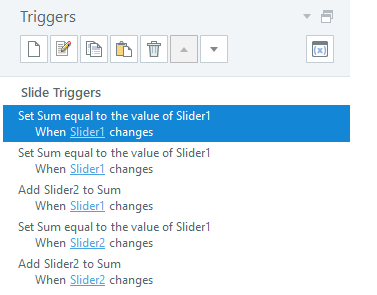# Intersecting sliders

Hi all,

I'm building a risk matrix that displays a different risk level depending on where you position two sliders (consequence x likelihood). The resulting risk level depends on both factors and I'm trying to create triggers to make that work. I've given each slider a variable and each position a value. If I could create another variable that would add the two slider variables together each time the slider moved then I could create a range of values that would display each risk level. I don't seem to be able to do this because that variable won't work, it won't assign a value of two other variables added together - any ideas?

Screenshots of matrix plus sliders attached.

###### 3 RepliesHi Amy,

Because you have 5 values for each you can use the 10s digit for the 2nd sllider. Set the 2nd slider to have values ranging from 10 to 50, with steps of 10. Create a new variable for the sum. Below, I named it Sum (creative, right?) Each time one of the slider variables changes, assign the sum variable first to Slider1, then add Slider2 to the sum. The sum variable is then cleared and recalculated with each change. You will have 25 possible values of that variable: 11, 12, 13, 14, 15, 21, 22, 23... through to 51, 52, 53, 54, 55. Then you'll have a value for each possible box in your matrix.